SCERT AP 7th Class Maths Solutions Pdf Chapter 12 Symmetry InText Questions and Answers.

## AP State Syllabus 7th Class Maths Solutions 12th Lesson Symmetry InText Questions

[Page No. 99]

Look at the following picture and speak about what you have noticed regarding symmetry in the picture.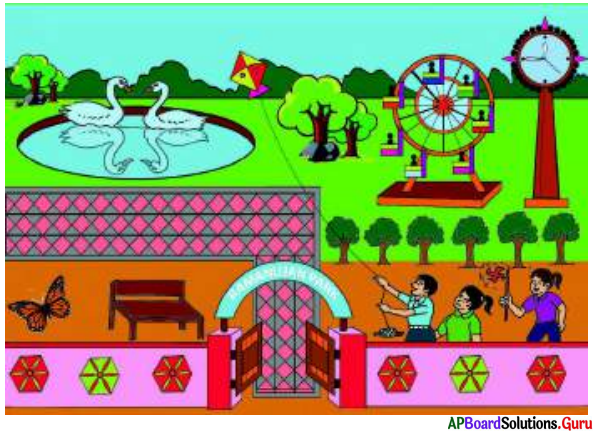Question 1.
What do you observe in this picture?
Ducks have symmetry.
Wall paintings have symmetry.
Butterfly has symmetry.
Clock tower and gaint wheels have symmetry.

Question 2.
Can you name the different shapes in picture?
Hexagon, Circle, Rectangle, etc.

Question 3.
Which figures are looking attractive and beautiful? Why?
Flooring, Gate arch and wall painting are beautiful as they have lines of symmetry.Question 4.
Which figures are in symmetry?
Ducks, wall paintings, gaint wheel and the clock tower have symmetry.

Question 5.
Can you draw line(s) of symmetry for them?
Yes.

[Page No. 100]

Look at the following figures. If we fold them exactly to their equal halves, one half of each figure exactly coincides with other half.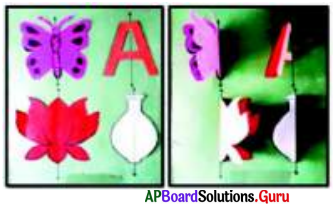Question 1.
What do we call such figures?
Symmetric figures or shapes.

Question 2.
What do we call the line along which we fold the figures so that, one half coincides with the other?
Line of symmetry.

Let’s Explore [Page No.102]

Question 1.
Find relation between the number of sides of regular polygon and its lines of symmetry.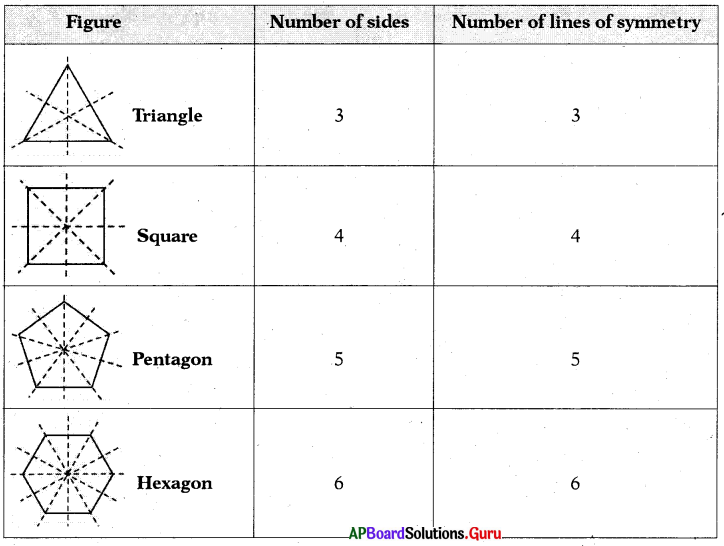From the above table, we conclude that the number of sides of a regular polygon is equal to its number of lines of symmetry.Question 2.
How many lines of symmetry can be drawn to a circle?
Number of lines of symmetry can be drawn to a circle are infinite.

Let’s Think [Page No. 103]

Question 1.
Draw any three shapes basing on below sentences :
(i) no line of symmetry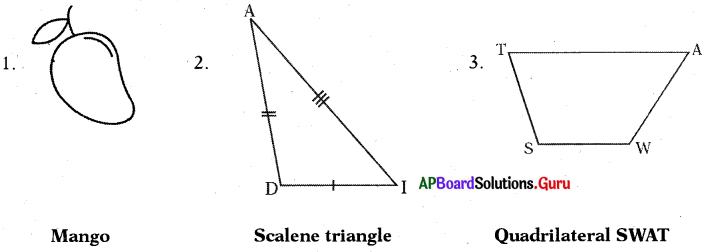(ii) one line of symmetry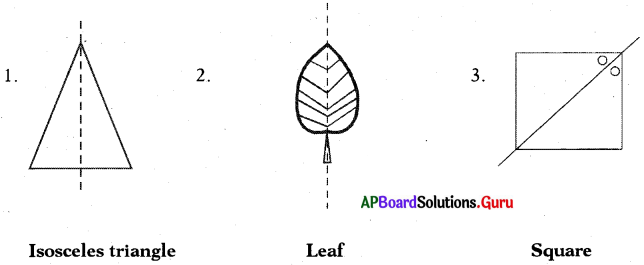(iii) two lines of symmetry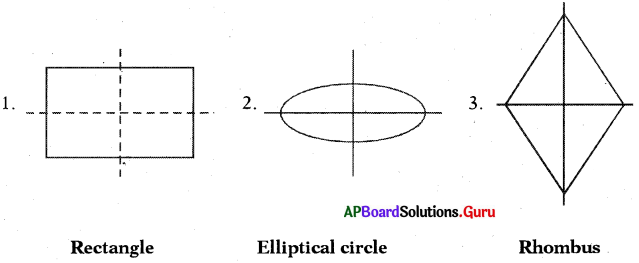(iv) three lines of symmetry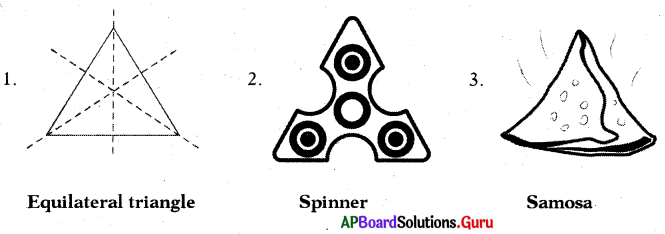Check Your Progress [Page No. 105]

Find whether the following letters of the English alphabet have rotational symmetry or not. If yes, find the point of rotational symmetry (approximately), and also order of rotational symmetry.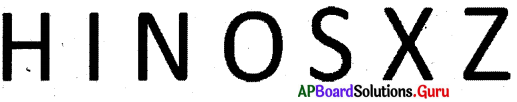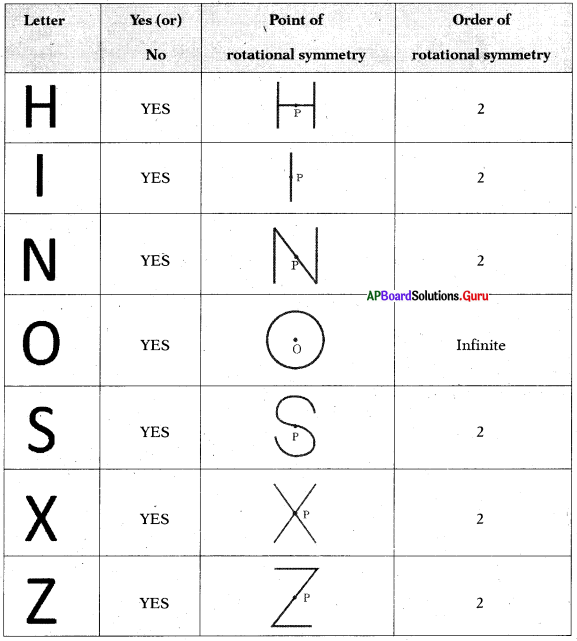Check Your Progress [Page No. 107]

Look at the following pictures and write their angle of symmetry and order of symmetry.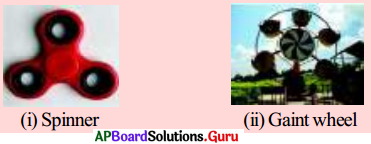(i) Spinner: Angle of symmetry = 120°
Order of symmetry = 3

(ii) Gaint wheel : Angle of symmetry = 60°
Order of symmetry = 6[Page No. 108]

Tesselations: The majority of the objects what we use in daily life have atleast one type of symmetry. Most of the machine made products are symmetric.

Observe these patterns :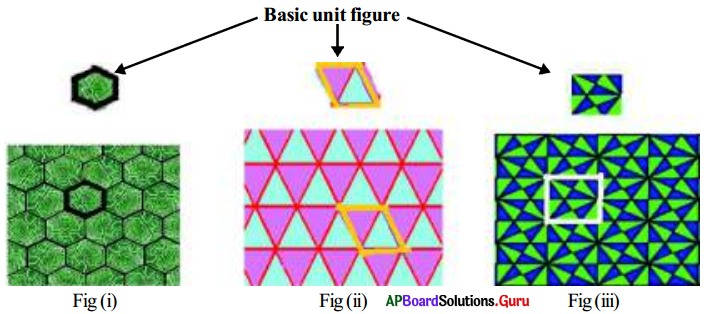(i) Where do you see these?
We see these patterns in floor designs and fabric painting etc.

(ii) How these patterns are formed? Are they symmetric as whole?
Does the basic figure which is used to form the tessellations symmetric?
You can observe that only some patterns have symmetry as a whole as in fig(i) and (ii), through the basic figures/unit figures are symmetric. Observe the fig(iii). You may notice that the two shapes can observe as ‘Basic or unit figures’ which is square or hexagon used to draw tessellation.

Usually, these patterns are formed by arranging congruent figures side by side in all the directions to spread upon an area without any overlaps or gaps. This is called tessellation. This enhances the beauty of the diagrams.

Let’s Explore [Page No. 109]

Fill the following with different colours to get beautiful tessellation patterns.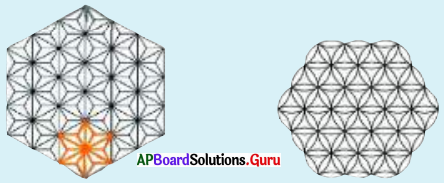Not needed.
Student should colour it on his own.

Let’s Explore [Page No. 109]

Construct a square having side 3cm. Draw all possible lines of symmetry. (Steps of construction is not necessary)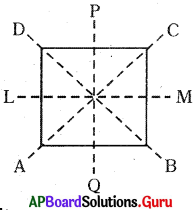1. Let ▢ABCD be the square with side 3 cm.
2. AC, BD, LM and PQ are lines of symmetry.

Examples:

Question 1.
If an equilateral triangle ABC is rotated through 360° about the point of rotation ‘P’ (i.e., point of intersection of angle bisectors), it attains the original form three times upon rotation through 120°, 240° and 360° as shown below.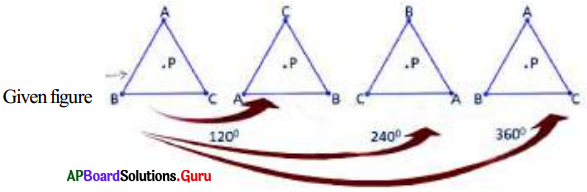Clearly, the above figure possesses a rotational symmetry of order 3.Question 2.
While rotating the following figure through 360° about the point ‘O’ (mid-point of BC), it attains the original form twice (upon rotation through 180° and 360°) as shown below.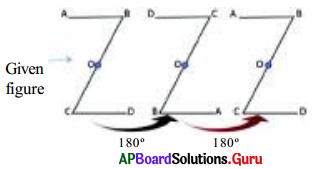Clearly, the above given figure possesses a rotational symmetiy of order 2.

Question 3.
Check whether the letter ‘S’ has point symmetry.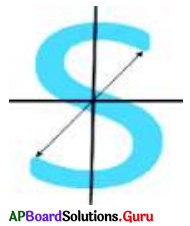Yes, it has a point symmetry.
Because, in the given figure, we have
i) Every part of the letter has a matching part are at the same distance from the central point.
ii) The part of the alphabet and its matching part are in the opposite direction.

[Page No- 111]

The image of an object as seen in a mirror is known as mirror image./In mirror image of an object, right side of the object appears at left side and vice versa. There are some objects whose mirror images are identical to the objects. For example, 11 capital English alphabets whose mirror images remain unchanged. They are A, H, I, M, O, T, U, V, W, X and Y.

Mirror Images of English Alphabets and some numbers :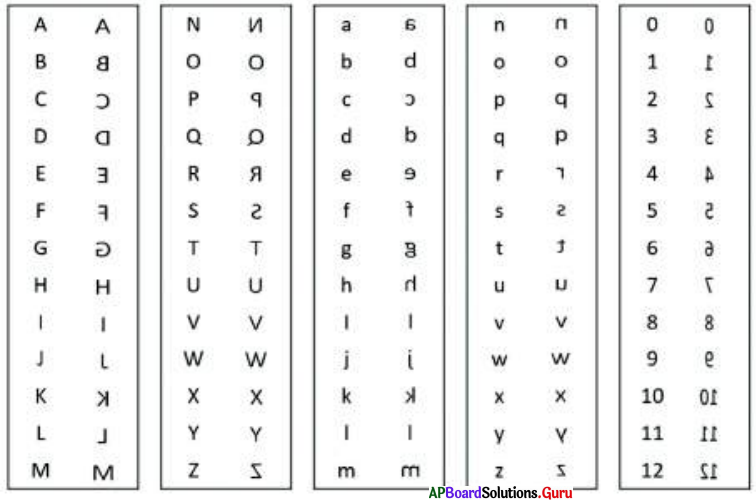Practice Questions [Page No. 112]

Choose the correct mirror image of the given words.

Question 1.
LATERAL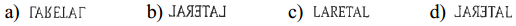(b)

Question 2.
QUANTITATIVE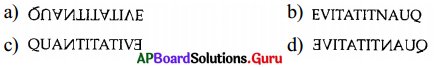(d)

Question 3.
JUDGEMENT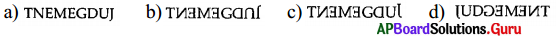(c)Question 4.
EMANATE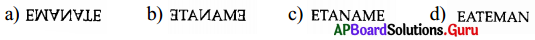(b)

Question 5.
KALINGA261B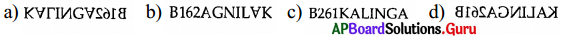(d)

Question 6.
COLONIAL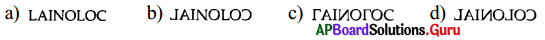(d)

Question 7.
BR4AQ16HI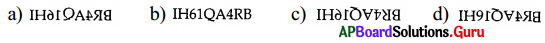(a)

Question 8.
R4E3N2U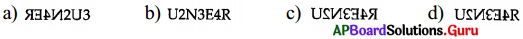(c)

Question 9.
DL3N469F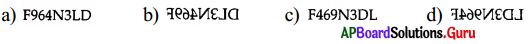(b)

Question 40.
MIRROR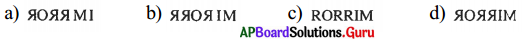(d)

Water images [Page No. 112]

The reflection of an object as seen in water is known , as water image. The upper part of the object seen downward and vice-versa. There a re some objects whose water images are identical to the objects. For example, 9 cap ital English alphabets given below, whose water images remain unchanged. They ar e B, C, D, E, H, I, K, O and X.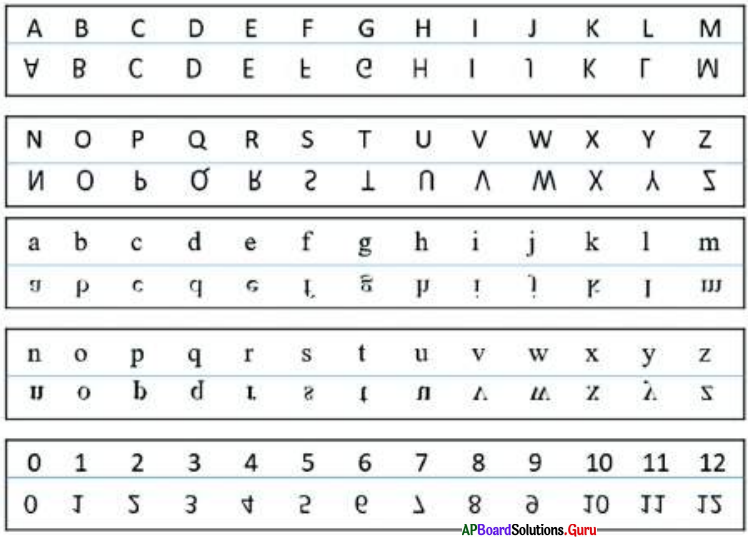Practice Questions [Page No. 113]

Choose the correct water image of the given words.

Question 1.
KICK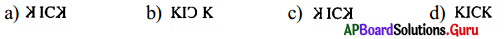(d)

Question 2.
UPKAR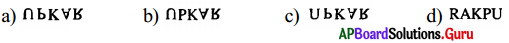(a)

Question 3.
KID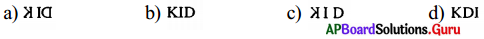(b)

Question 4.
SUBHAM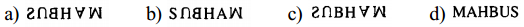(c)

Question 5.
CHIDE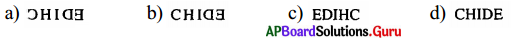(d)

Question 6.
HIKE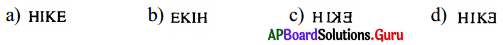(a)

Question 7.
COT5E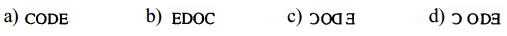(a)Question 8.
ab45CD67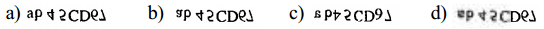(b)

Question 9.
abc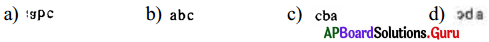(a)

Question 10.
01234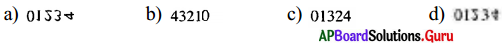(a)

Mirror image of the clock [Page No. 114]

1. A clock is a device provided with three hands an hour hand, minute hand and second hand.
Hours hand also known as short hand and minutes hand is also known as long hand.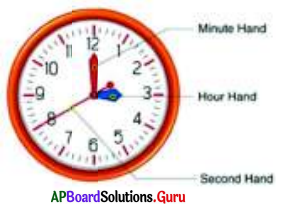2. The face of the clock is divided into 12 equal divisions.
The angular space between any two consecutive divisions has further divided into five more divisions.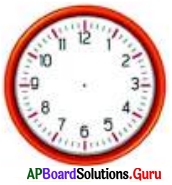Let us observe the following images of a clocks and their mirror images :
Case I: To find the time in the mirror image subtract the actual time from 11 hours 60 minutes.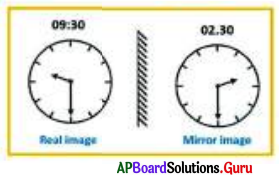Example 1:
If actual time in clock is 9 hours 30 minutes then, what is’the time shown in mirror?
11 hrs 60 min – 09 hrs 30 min – 2 hrs 30 minExample 2 :
If actual time in clock is 7 hours, then what is the time shown in mirror?
11 hrs 60 min – 07 hrs 00 min = 4 hrs 60 min = 5 hrs (1 hour — 60 minutes)

Case II : If the time in clock is between 12 hrs and 01 hr then to find the time in the mirror image subtract the actual time from 23 hr 60 min.
Example :
If actual time in clock is 12 hours T5 minutes, then what is the time shown in mirror?
23 hrs 60 min — 12 hrs 15 min =11 hrs 45 min

Practice Questions [Page No. 114]

Choose the correct mirror image of the given words.

Question 1.
If actual time in dock is 06 hours 10 minutes, then what is the time shown in mirror?
(a) 3 hr:s 50 min
(b) 4 hrs 50 min
(c) 5 hrs 50 min
(d) 6 hrs 50 min
(c) 5 hrs 50 min

Question 2.
If actual time in clock is 03 hours 54 minutes, then what is the time shown in mirror?
(a) 8 hrs 06 min
(b) 9 hrs 06 min
(c) 8 hrs 54 min
(d) 9 hrs 54 min
(a) 8 hrs 06 min

Question 3.
If actual time in clock is 08 hours 26 minutes, then what is the time shown in mirror?
(a) 6 hrs 34 min
(b) 3 hrs 34 min
(c) 1 hr 34 min
(d) 3 hrs 36 min
(b) 3 hrs 34 minQuestion 4.
If actual! time in clock is 4 hours, then what is the time shown in mirror?
(a) 7 hrs
(b) 7 hrs 30 min
(c) 8 hrs
(d) 8 hrs 30 min
(c) 8 hrs

Question 5.
If actual time in dock is 10’o clock, then what is the time shown in mirror?
(a) 2 hrs
(b) 3 hrs
(c) 4 hrs
(d) 5 hrs
(a) 2 hrs

Question 6.
If the time shown in mirror is 10 hours 05 minutes, then what is the actual time in clock?
(a) 1 hrs 55 min
(b) 1 hrs 35 min
(c) 1 hrs 25 min
(d) 12 hrs 15 min
(a) 1 hrs 55 min

Question 7.
If the time shown in mirror is 02 hours 47 minutes, then what is the actual time in clock?
(a) 6 hrs 13 min
(b) 7 hrs 13 min
(c) 8 hrs 13 min
(d) 9 hrs 13 min
(d) 9 hrs 13 min

Question 8.
If actual time in clock is 11 hours 45 minutes, then what is the time shown in mirror?
(a) 1 hrs 15 min
(b) 3 hrs 15 min
(c) 6 hrs 15 min
(d) 12 hrs 15 min
(d) 12 hrs 15 min

Question 9.
If actual time in clock is 12 hours 45 minutes, then what is the time shown in mirror?
(a) 9 hrs 15 min
(b) 10 hrs 15 min
(c) 11 hrs 15 min
(d) 12 hrs 15 min